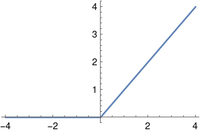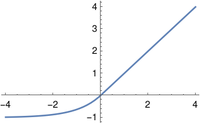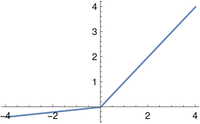# Activation functions and Iverson brackets

Neural network activation functions transform the output of one layer of the neural net into the input for another layer. These functions are nonlinear because the universal approximation theorem, the theorem that basically says a two-layer neural net can approximate any function, requires these functions to be nonlinear.Activation functions often have two-part definitions, defined one way for negative inputs and another way for positive inputs, and so they’re ideal for Iverson notation. For example, the Heaviside function plotted above is defined to beKenneth Iverson’s bracket notation, first developed for the APL programming language but adopted more widely, uses brackets around a Boolean expression to indicate the function that is 1 when the expression is true and 0 otherwise. With this notation, the Heaviside function can be written simply asIverson notation is fairly common, but not quite so common that I feel like I can use it without explanation. I find it very handy and would like to popularize it. The result of the post will give more examples.

## ReLUThe popular ReLU (rectified linear unit) function is defined asand with Iverson bracket notation asThe ReLU activation function is the identity function multiplied by the Heaviside function. It’s not the best example of the value of bracket notation since it could be written simply as max(0, x). The next example is better.

## ELUThe ELU (exponential linear unit) is a variation on the ReLU that, unlike the ReLU, is differentiable at 0.The ELU can be described succinctly in bracket notation.## PReLUThe PReLU (parametric rectified linear unit) depends on a small positive parameter a. This parameter must not equal 1, because then the function would be linear and the universal approximation theorem would not apply.In Iverson notation:## One thought on “Activation functions and Iverson brackets”

1. Hey John,

there is another activation function, instead of 50% scale invariant like the ReLU function, the Reflected ReLU offers 100%. For this you need a new equivalent set of weights. For positive activation values, the positive weights, which can be + or -. And for negative activations, the negative weights, which can also be + or -, whatever it takes. This leads to 2 dimensional weights.

How would you note the Reflected ReLU with Iverson brackets?

It is questionable how wise this is, even if really good results can be achieved due to the larger bandwidth, but a larger ReLU layer with the same parameters then seems to be perhaps the better choice. ReLU can close dimensions and maintain positive continuous value like no other activation function, so ReLU FTW!

Also ReLU can be pre-activated, which means to skip ~80% computations on each layer. https://github.com/grensen/neural_network_benchmark#relu-pre-activation

for (int l = 0, w = 0; l 0)
for (int r = 0; r < right; r++) // output
neurons[r] += n * weights[w + r];
w += right;
}

How would you write this?

Iverson brackets seem to be the more natural way to go, great article.